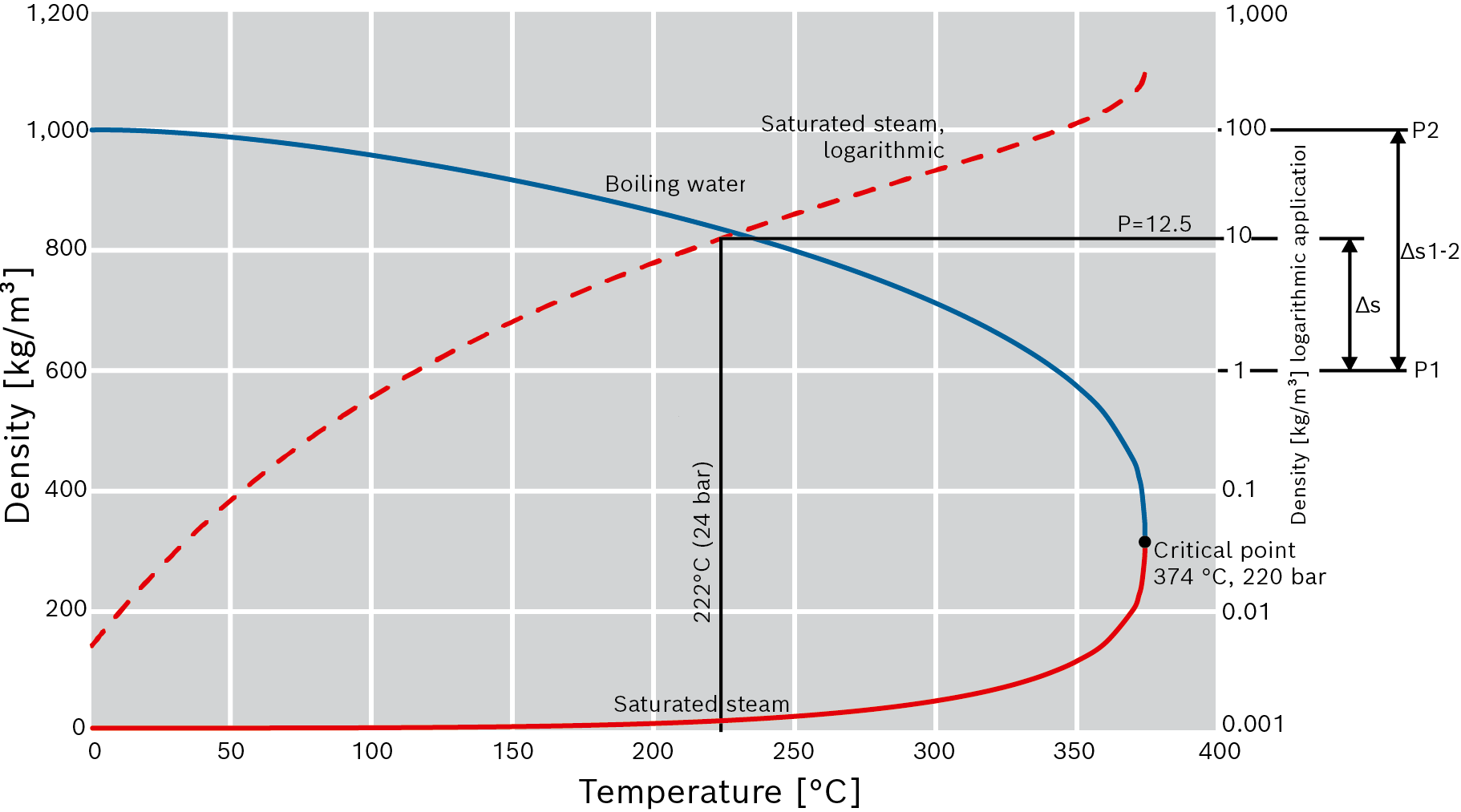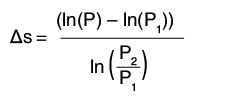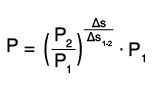## DensityDensity of saturated steam and water as a function of temperatureBoiling waterSaturated steam, logarithmicSaturated steamExample, saturated steam density From value to distance in the graph Units in the example 1): First point on the logarithmic scale P1 kg/m³ Second point on the logarithmic scale P2 kg/m³ Distance between the two points ∆s1-2 mm Required point P kg/m³ Distance between P1 and P ∆s 14.86 mmFrom the distance in the diagram to the value First point on the logarithmic scale P1 kg/m³ Second point on the logarithmic scale P2 kg/m³ Distance between the two points ∆s1-2 mm Required point P 12.47 kg/m³ Distance between P1 and P ∆s mm1) The conversion can be applied to all logarithmic diagrams irrespective of the units